HTML Tutorial: Lines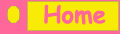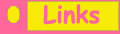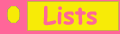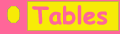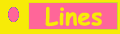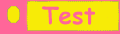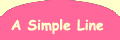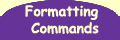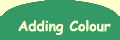## The Basic Line : <HR>

This is a simple, but very effective command to use - it creates a horizontal line, with a little shading which is centred across the page, like this:

It is very useful for dividing a page into sections. By the way HR stands for Horizontal Reference.

Is that all there is to it? Well yes - there aren't any start or end tags, however, there are some attributes you can  use to jazz it up a bit .......

## Changing The Width

You change the width of a line by using the width attribute - the  following commands made the lines above (note you need to use the % sign).

• <hr width = 80%>
• <hr width = 60%>
• <hr width = 40%>

Top of Page

## Changing The Height

Thicker lines are made with the following commands:



The SIZE attribute = height.

Top of Page

## Aligning the Line

Here I used the ALIGN attribute - the following four commands made the above lines:

<HR WIDTH="60%" ALIGN="LEFT">
<HR WIDTH="60%" ALIGN="RIGHT">

<HR WIDTH="60%" ALIGN="LEFT">
<HR WIDTH="60%" ALIGN="RIGHT">

Whilst you can say ALIGN="CENTER" its a bit of an overkill as this is the default i.e. what the attribute is set to if you don't specify left or right.

Top of Page

Easily done with the color attribute - note I'm also using the WIDTH attribute to make lines which span just 60%.

<HR COLOR="yellow" WIDTH="60%">
<HR COLOR="purple" WIDTH="60%">
<HR COLOR="green" WIDTH="60%">

## Making a Solid Line

If you don't want any shading but a solid line instead then this is the attribute for you ...

<HR COLOR="pink" WIDTH="60%" SIZE="15" NOSHADE>

Try it out yourself in the box below: